Back

## Canine Chaos

Aditya, Basil, Cecilia, and Dimitri each teach one class at $\text{8:00},$ $\text{9:00},$ $\text{10:00},$ or $\text{11:00}.$ At those times, they each teach algebra, calculus, geometry, or probability. Everybody teaches at a different time, and everybody teaches a different class.

For each teacher, we’d like to know when they teach and which course they teach. Given a set of clues, how can we figure it out?

These are the makings of a logic grid puzzle. To work through this problem, keep reading. Or, skip to the challenge for a puzzle of your own.

Here are the clues:

1. One of Basil's class and the $\text{9:00}$ class is calculus and the other is geometry.
2. The four classes are Cecilia’s, the $\text{10:00}$ class, geometry, and probability.
3. Aditya’s class is one hour before calculus.
4. Calculus is two hours before geometry.$\\[-0.7em]$

The information is hard to make sense of in this form — most of the clues involve information relating to all three categories (teacher, class time, and course). It's easier to interpret the clues if we use an organizational tool that allows us to track the relationships by two categories at a time. $\\[-1em]$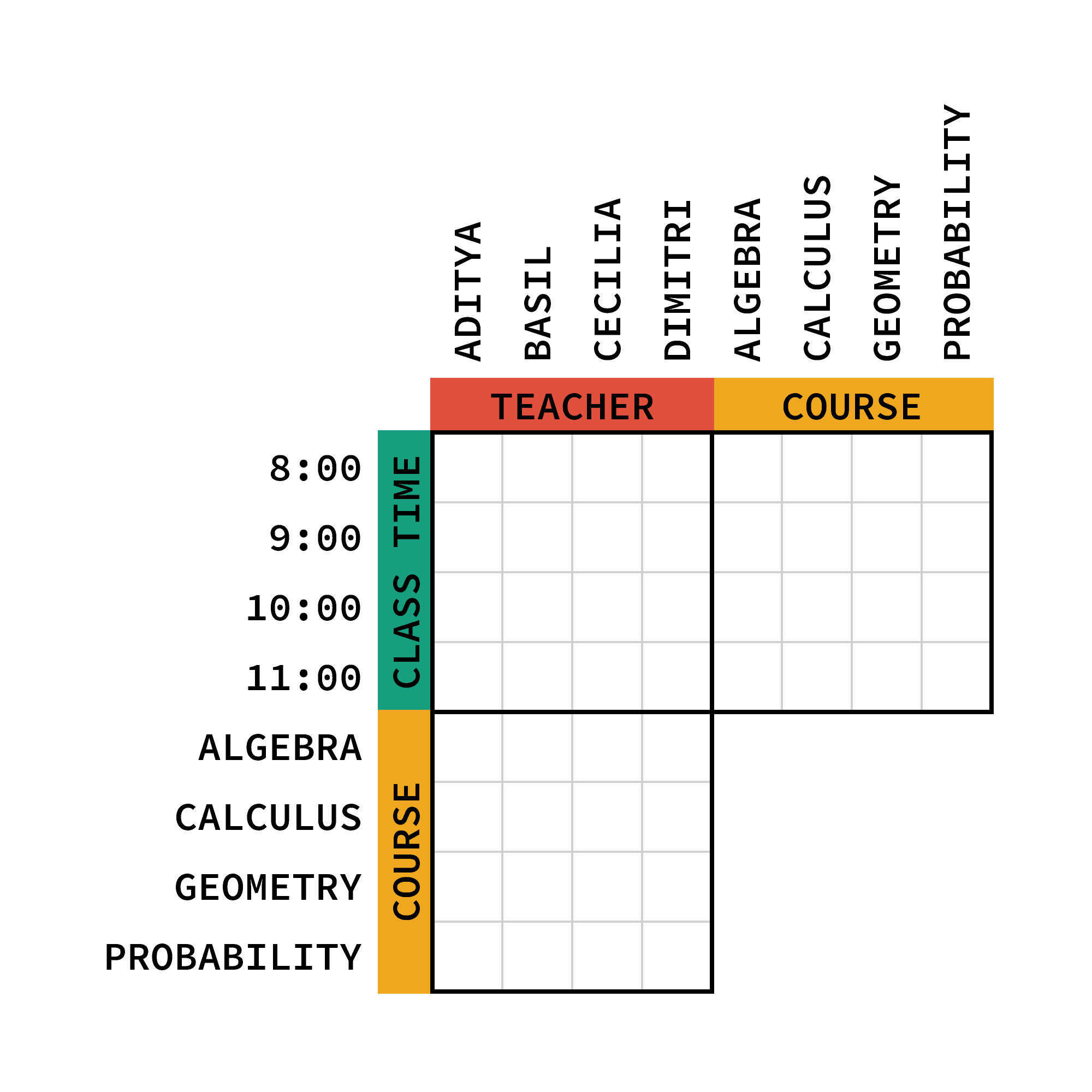$\\[-1em]$ The grid has three large regions, one for each pairing of categories — teacher and class time, teacher and course, and class time and course. In each region, there is a square for each possible pair of attributes within those two categories. We can mark up the grid when we know that a pair of attributes does or does not make a match.

First, let's go through and eliminate every pair that we can, based on the clues given.

Clue 1 implies that Basil's class is different from the $\text{9:00}$ class. Additionally, both Basil's class and the $\text{9:00}$ class can only be calculus or geometry.

Clue 2 tells us that the four attributes listed are for different classes. That is, Cecilia's class isn't at $\text{10:00},$ isn't geometry, and isn't probability. Also, the $\text{10:00}$ class isn't geometry or probability.

Clue 3 means that Aditya doesn't teach calculus. We also get that calculus can't be at $\text{8:00}$ since there's no class one hour earlier. Similarly, Aditya's class can't be at $\text{11:00}$ because there's no class one hour later.

Finally, clue 4 means calculus can only be at $\text{8:00}$ or $\text{9:00},$ and geometry can only be at $\text{10:00}$ or $\text{11:00}.$

$\\[-1em]$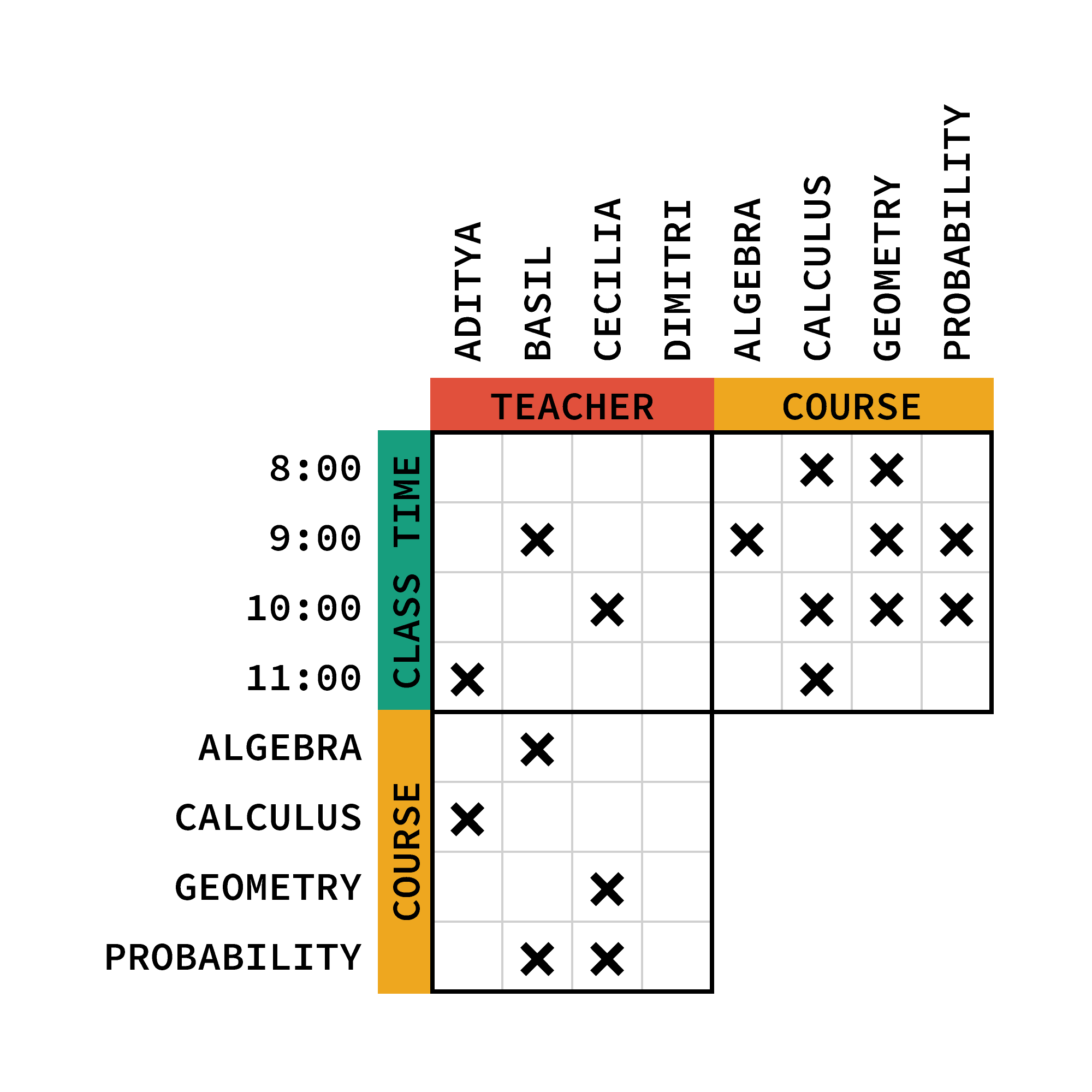$\\[-1em]$ At this point, from the grid, we can see that the class times for all of the courses are forced:

Calculus must be at $\text{9:00},$ and geometry must be at $\text{11:00}.$ The only course that can take place at $\text{10:00}$ is algebra. Then probability must be the $\text{8:00}$ class.

Furthermore, since Aditya's class is one hour before calculus (clue 3), she must teach at $\text{8:00}.$ That means Aditya teaches probability.

$\\[-1em]$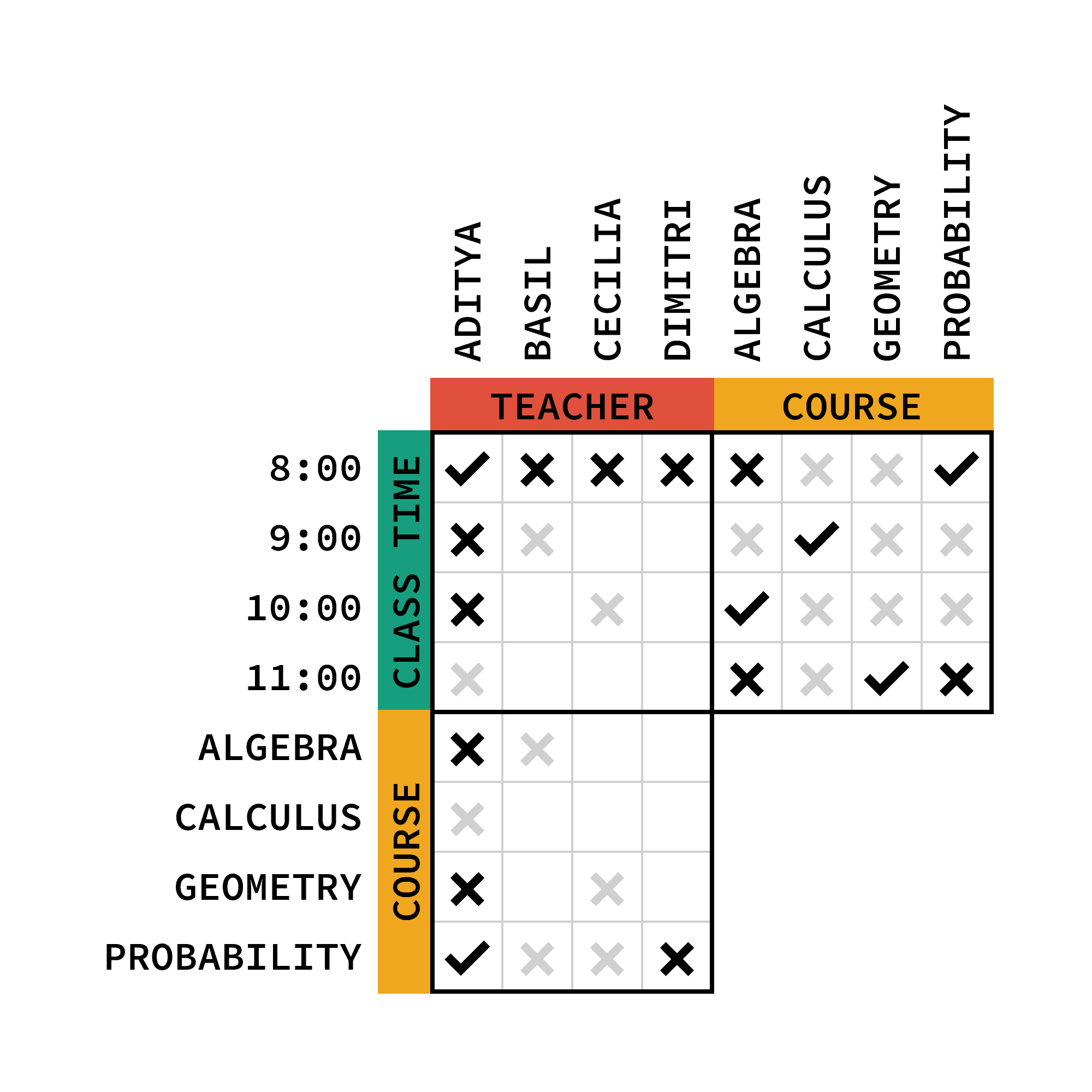$\\[-1em]$ When we place a check mark in the grid, it represents a match between attributes. Within each region of the grid, each row and column can only have one check mark.

Since Aditya teaches at $\text{8:00},$ none of the other teachers can. Additionally, Aditya cannot teach at any of the other times. Similarly, since Aditya teaches probability, none of the other teachers can, and Aditya cannot teach any of the other courses.

With the class time and course region of the grid complete, we can transfer some of that information to other regions.

Basil can only teach at $\text{10:00}$ or $\text{11:00},$ so he can only teach algebra or geometry. We already found that he doesn't teach algebra, so he must teach geometry. Geometry is at $\text{11:00},$ so Basil teaches at $\text{11:00}.$

$\\[-1em]$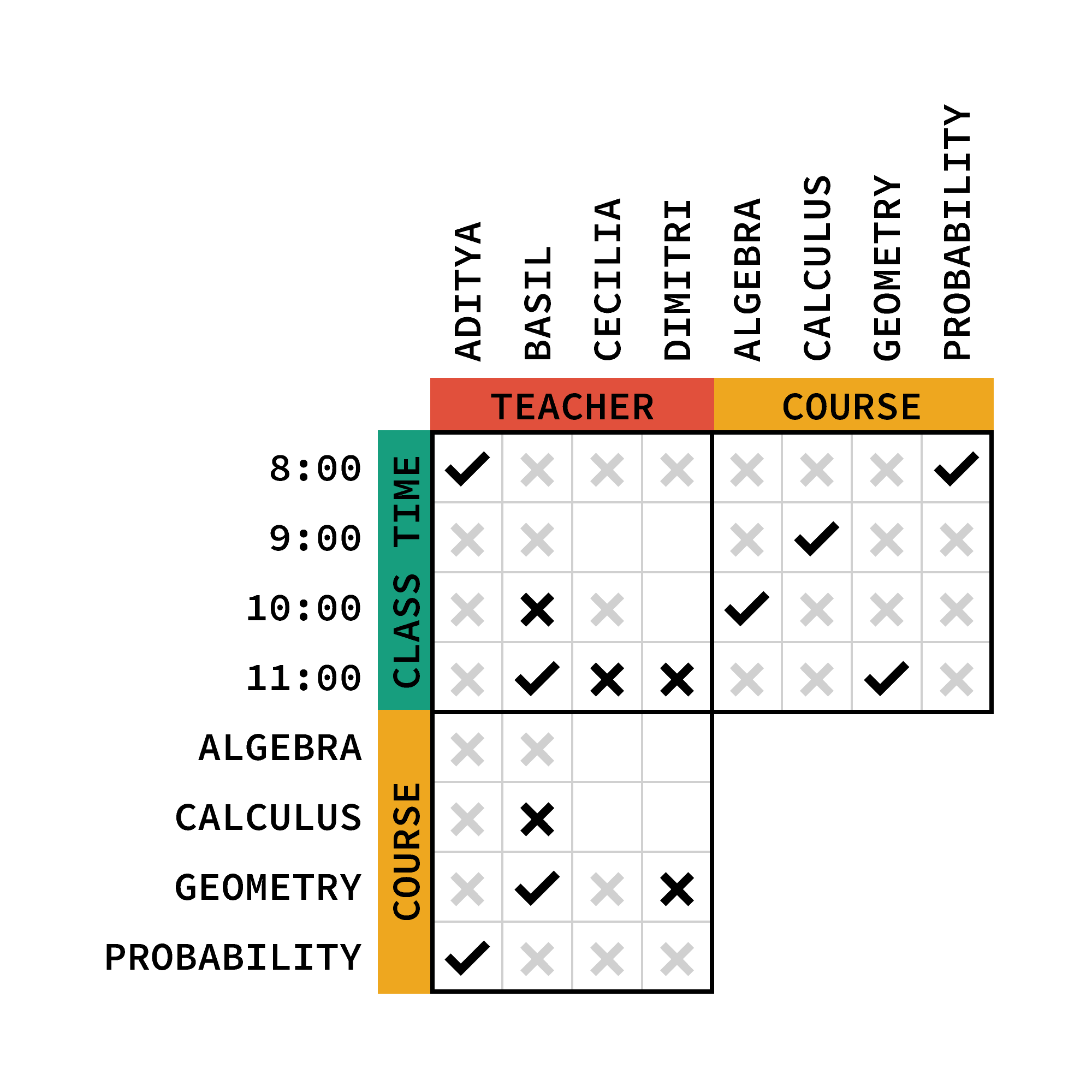$\\[-1em]$ Everything else we need to know is forced:

The $\text{10:00}$ class has to be taught by Dimitri, so he teaches algebra. That leaves Cecilia to teach at $\text{9:00},$ so she teaches calculus.

$\\[-1em]$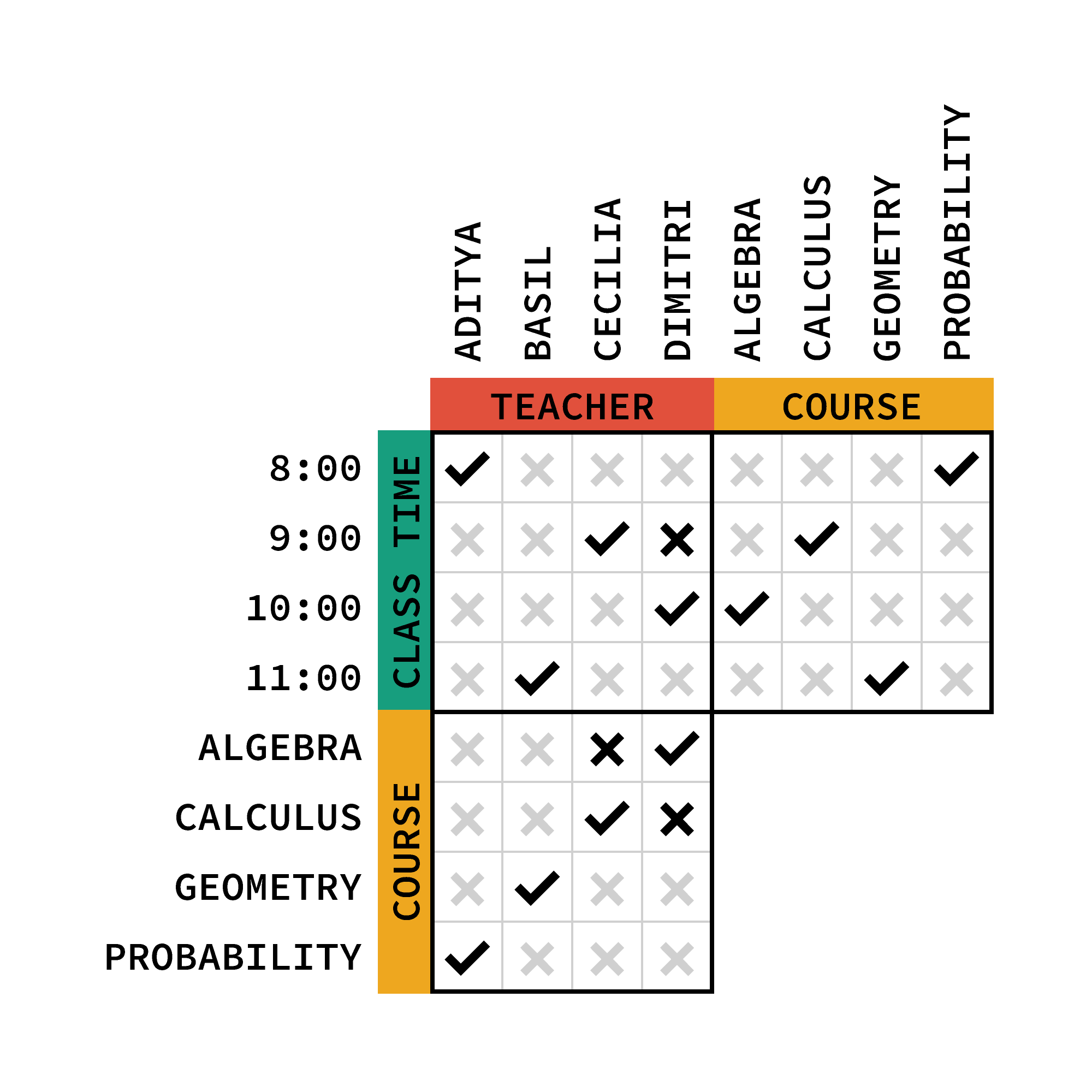$\\[-1em]$ Now that you’ve seen how to translate a set of clues to the grid, can you solve a logic grid puzzle of your own?

# Today's Challenge

Today at Brilliant Dog Shelter, the puppies named Agnes, Beatrice, Hubert, Rhapsody, and Tyrone all left for their forever homes!While updating their files, a new employee at the shelter mixed up their information and needs to get it all back in order. She knows that their breeds are retriever, corgi, dalmatian, bulldog, and dachshund and that they weigh $1, 2, 3, 4,$ and $5\text{ kg}.$

She also remembers these:

• Rhapsody is either the dalmatian or the $4\text{-kg}$ puppy.
• The corgi is $1\text{ kg}$ lighter than Rhapsody.
• The five puppies are the corgi, the $3\text{-kg}$ puppy, Hubert, the $1\text{-kg}$ puppy, and Agnes.
• One of Hubert and Beatrice is $5\text{ kg}$ and the other is the dachshund.
• Agnes isn't the bulldog.$\\[-0.6em]$

$\\[-0.4em]$ What kind of dog is Tyrone?

$\\[-0.3em]$×

Problem Loading...

Note Loading...

Set Loading...